# Real closed field

An ordered fieldfor which no non-trivial algebraic extension (cf. Extension of a field) can be ordered. E. Artin and O. Schreier ([a1]) characterized real closed fields to be the fieldsfor which the algebraic closure (cf. Algebraically closed field) is a non-trivial finite extension; in this case, it is of degreeand obtained by adjoining the square root of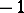. Every ordered fieldhas an algebraic extension which is real closed, unique up to order-preserving isomorphism over. It is called the real closure of.

## Model theory.

In 1940, A. Tarski proved that the elementary theory of all real closed fields is complete; this is known as the Tarski principle. It implies that, roughly speaking, Euclidean geometry remains the same if the fieldof real numbers is replaced by any other real closed field, at least for those theorems which can be formulated in a first-order language of ordered fields (and these are many geometrical theorems). This is the real analogue of the elementary version of the Lefschetz principle (cf. Transfer principle).

Tarski also proved that the elementary theory of real closed fields admits elimination of quantifiers (cf. [a11]). In 1956, A. Robinson deduced model completeness by simple model-theoretic techniques from the uniqueness of the real closure and the fact that the order of an extension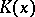of a real closed fieldwith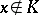is uniquely determined by the cut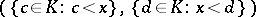thatinduces in. This can be adapted to prove elimination of quantifiers.

The theory of real closed fields can be recursively axiomatized (cf. Axiomatized class) in a first-order language of ordered rings or fields (cf. Structure). To the axioms for ordered fields, one only has to add an infinite axiom scheme expressing the sign change property, which says that every polynomial must have a root in a given interval where it changes sign; forthis is the content of the intermediate value theorem (cf. Cauchy theorem). Since the theory is complete, the recursive axiomatization yields decidability. The completeness also shows that all first-order theorems and axioms of Euclidean geometry are consequences of the axioms given in this axiomatization.

Further, it follows from work of G.L. Cherlin and M. Dickmann (1983) that the theory of real closed fields with a convex valuation ring admits elimination of quantifiers in the language of ordered fields enriched by a unary relation symbol for being an element of the valuation ring (cf. Model theory of valued fields).

## Hilbert's 17th problem and a real Nullstellensatz.

Artin gave a positive answer to Hilbert's 17th problem in [a1], using a generalization of the Sturm theorem. He proved that ifis real closed,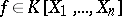and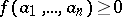for all, thenis a sum of squares in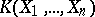. (Note that the squares have to be taken over elements of the rational function field.) Hilbert's original 17th problem asks for an analogous result withreplaced by the field of rational numbers; the above also holds for this case sincecan be replaced by any ordered field which is dense in its real closure and admits a unique order.

The theorem was reproved by A. Robinson in 1955 using model completeness, which can also be used to prove a real version of Hilbert's Nullstellensatz (cf. Hilbert theorem). There are many such versions, due to several authors. The following is known as the Dubois Nullstellensatz (D.W. Dubois, 1969): Ifis an ordered field,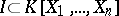an ideal andsuch that every common root of all polynomials inin the real closure ofis also a root of, thenlies in the real radical of. The real radical is defined to be the set of allfor whichfor some, where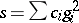is a sum of squares of elements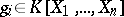with positive coefficients.